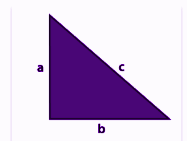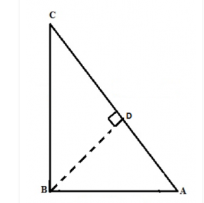# Hypotenuse

In Mathematics, the term “Hypotenuse” comes from the Greek word hypoteinousa that means “stretching under”. This term is used in Geometry, especially in the right angle triangle. The longest side of a right-angle triangle is called the hypotenuse (the side which is opposite to the right angle). In this article, we are going to discuss the meaning of the term hypotenuse along with its formula, theorem, proof and examples.

## Hypotenuse Meaning

Hypotenuse means, the longest side of a right-angled triangle compared to the length of the base and perpendicular. The hypotenuse side is opposite to the right angle, which is the biggest angle of all the three angles in a right triangle. Basically, the hypotenuse is the property of only the right triangle and no other triangle. Now, this is better explained when we learn about the right-angled theorem or Pythagoras Theorem or Pythagorean theorem. These concepts are majorly used in Trigonometry. Here, in this article, we will learn in detail about hypotenuse, its formula, theorem along with examples.

## Hypotenuse Theorem

The hypotenuse theorem is defined by Pythagoras theorem, According to this theorem, the square of the hypotenuse side of a right-angled triangle is equal to the sum of squares of base and perpendicular of the same triangle, such that;

Hypotenuse2 = Base2 + Perpendicular2

## Hypotenuse Formula

The formula to find the hypotenuse is given by the square root of the sum of squares of base and perpendicular of a right-angled triangle. The hypotenuse formula can be expressed as;

Hypotenuse = √[Base2 + Perpendicular2]

Let a, b and c be the sides of the triangle as per given figure below;So the hypotenuse formula for this triangle can be given as;

c2 = a2 + b2

Where a is the perpendicular, b is the base and c is the hypotenuse.

## Hypotenuse Theorem Proof

Given: A right triangle ABC, right-angled at B.

To Prove: Hypotenuse2 = Base2 + Perpendicular2Proof: In triangle ABC, let us draw a line from B to touch the side AC at D.

By similar triangles theorem, we can write;

Or AB2 = AD x AC ………………..1

Again, △BDC ~△ABC

So, CD/BC = BC/AC

Or

BC2 = CD x AC ……………2

Now, if we add eq. 1 and 2 we get;

AB2 + BC2 = (AD x AC) + (CD x AC)

Taking AC as common term from right side, we get;

AB2 + BC2 = AC (AD + CD)

AB2 + BC2 = AC (AC)

AB2 + BC2 = AC2

Base2 + Perpendicular2 = Hypotenuse2

Hence, proved.

## Hypotenuse of a triangle

The hypotenuse is only defined for the right-angled triangle. It is not defined for any other types of triangles in geometry such as

• Acute Angled Triangle
• Obtuse Angled Triangle
• Scalene Triangle
• Isosceles Triangle
• Equilateral Triangle

But only the isosceles triangle could be represented as a right-angled triangle, where the length of the base side and perpendicular side are equal and the third side will be the hypotenuse.

## How to Find the Altitude on a Hypotenuse?

In Maths, the altitude of a triangle means the line segment that connects the vertex and the side opposite to the vertex. The length of the altitude is simply called the “altitude”. Similarly, the altitude to the hypotenuse is the line segment that connects the hypotenuse of a right triangle and the vertex opposite to the hypotenuse through the perpendicular. In elementary geometry, the relationship between the length of the altitude on the hypotenuse of a right triangle and the line segment created on the hypotenuse is explained using the theorem called the “Geometric Mean Theorem” or “Right Triangle Altitude Theorem”. The altitude to the hypotenuse can be found as follows:

Step 1: In a right triangle, draw the altitude of the hypotenuse. The altitude creates the two new right triangles which are similar to each other and the main right triangle.

Step 2: Now, divide the length of the shortest of the main right triangle by the hypotenuse of the main right triangle.

Step 3: Now, multiply the result obtained from step 2 by the remaining side of the main right triangle.

Step 4: The result obtained is called the altitude or the height of the right triangle.

For example, if the sides of a right triangle a, b, and c are 3 cm, 4 cm,  and 5 cm respectively, then the altitude on the hypotenuse is calculated as follows:

Here

a = perpendicular side = 3 cm

b= base side = 4 cm

C = hypotenuse = 5 cm

Here, the smallest side of the right triangle is equal to 3 cm.

Therefore, divide the perpendicular side by the hypotenuse, we get

Altitude = ⅗ = 0.6

Now, multiply the result by the base side of the right triangle.

Altitude = 0.6 x 4

Altitude = 2.4 cm

Therefore, the altitude on the hypotenuse of a right triangle is 2.4 cm.

Similarly, the altitude can be found using trigonometry. The angles on the smaller triangles are the same as the angles in the main right triangle. Thus, by using the trigonometry formulas, we can find the altitude.

### Hypotenuse Examples

Let us solve some examples based on the hypotenuse concept.

Example 1:

If the base and perpendicular of a right-angled triangle are 3cm and 4cm, respectively, find the hypotenuse.

Solution:

Given, base = 3cm and perpendicular = 4cm

By the hypotenuse formula, we know;

Hypotenuse = √(Base2 + Perpendicular2)

= √(32 + 42)

= √(9 + 16)

= √25

= 5cm

Hence, the length of the hypotenuse is 5cm.

Example 2:

For an isosceles right-angled triangle, the two smallest sides are equal to 10cm. Find the length of the longest side.

Solution:

The two equal sides of the isosceles right triangle are the base and perpendicular.

The longest side is the hypotenuse. Hence, by using the formula, we get;

Hypotenuse = √(Base2 + Perpendicular2)

H = √(102 + 102)

H = √(100 + 100)

H = √200

H = 10√2 cm

Keep visiting BYJU’S – The Learning App and download the app to learn all the important Maths-related concepts with ease.

## Frequently Asked Questions on Hypotenuse

### What is meant by hypotenuse?

In Maths, a hypotenuse is the longest side of a right triangle. In other words, the side opposite to the right angle is called the hypotenuse.

### What is the formula to calculate the hypotenuse?

The formula to calculate the hypotenuse of a right triangle is:
Hypotenuse = √[Base2 + Perpendicular2]

### How to calculate the altitude of a right triangle?

The altitude of a right triangle can be determined using the following steps:
Step 1: Divide the smallest side of the right triangle by the length of the hypotenuse.
Step 2: Multiply the result obtained from step 2 with the remaining side of the right triangle. The result is the altitude or the height of a right triangle.

### What is meant by the right triangle altitude theorem?

The right triangle altitude theorem or the Geometric theorem states that the altitude to the hypotenuse of the right triangle forms two congruent triangles which are similar to the original right triangle.

### How to find the hypotenuse of a right triangle?

The length of the hypotenuse of a right triangle can be found with the help of the Pythagoras theorem. The theorem states that the square of the hypotenuse is equal to the sum of the square of the other two sides.